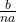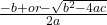## Carl is deriving the quadratic equation by completing the square. His work at one of the steps is shown. x + b/na = + or – b^2-4ac. Carl is

Question

Carl is deriving the quadratic equation by completing the square. His work at one of the steps is shown. x + b/na = + or – b^2-4ac. Carl is not sure what the value of n is. What value should he use to continue deriving the quadratic equation correctly? A. 1 B. 2 C. 3 D. 4

in progress 0
5 months 2021-08-02T20:59:36+00:00 1 Answers 8 views 0

## Answers ( )

the correct option is B. 2

Step-by-step explanation:

As given,

x += ± √b²-4ac

⇒x = –± √b²-4ac

As e know that the quadratic formula is

x =So , by comparing with the formula

we get

n = 2

So, the correct option is B. 2